tip

## 背景

`Google Bard AI`已经发布有一段时间了，我来深度体验一下，看一下它和ChatGPT谁更强，Google AI能不能实现弯道超车，巩固自己的地位。## 体验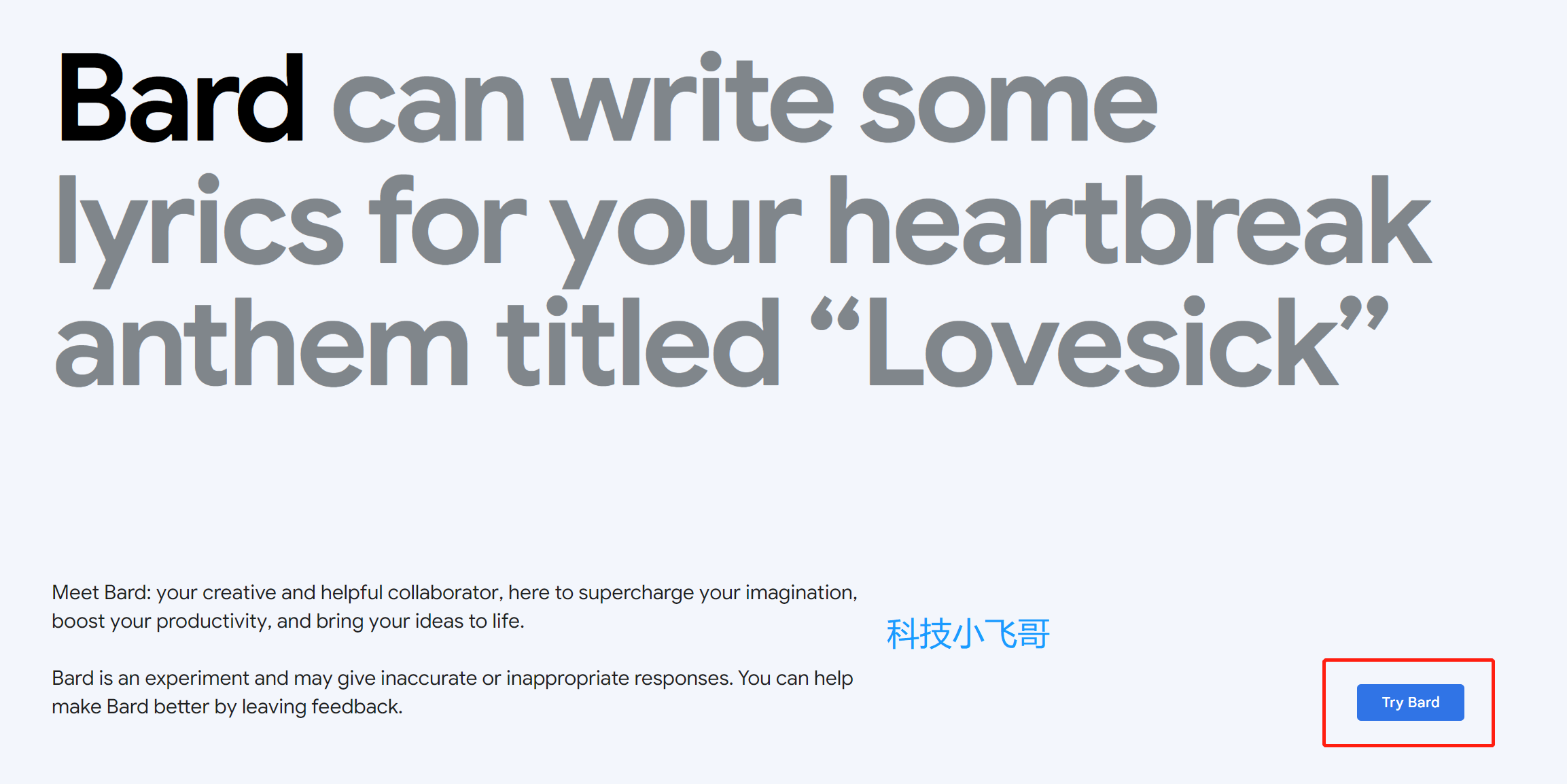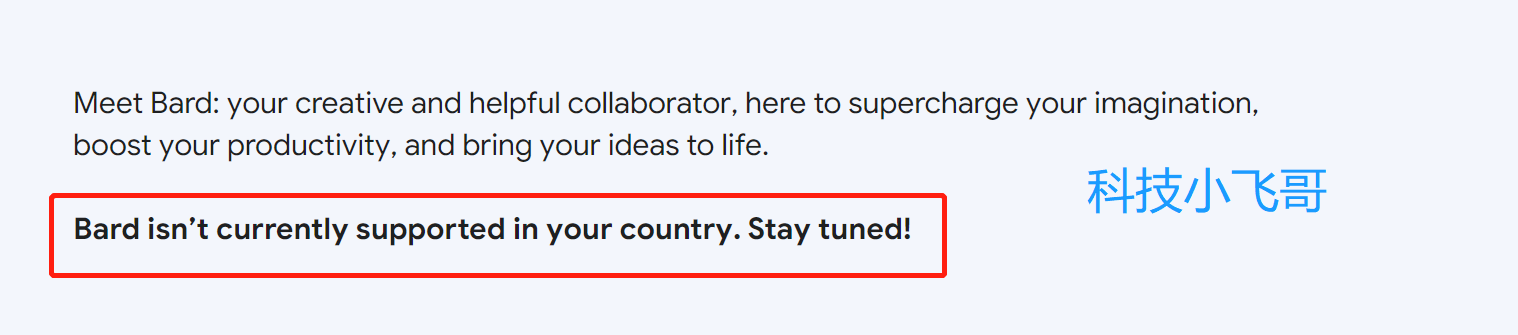### Q1: 你好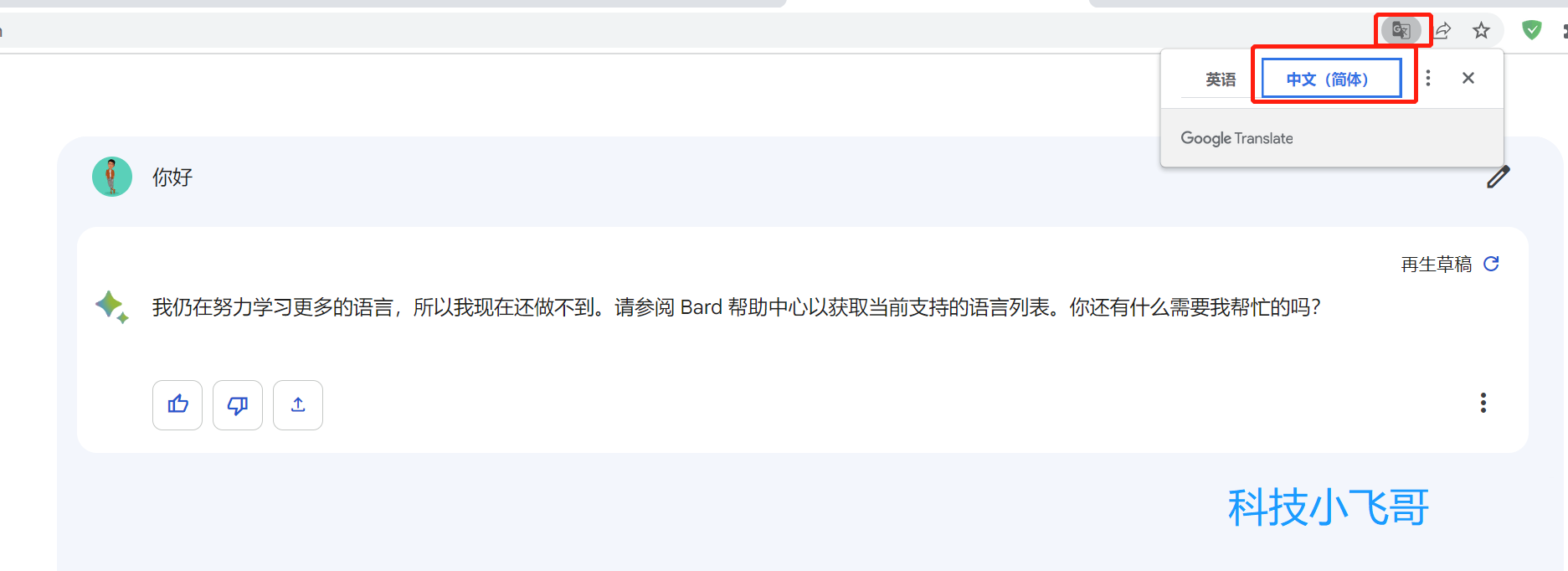### Q2: what happend today?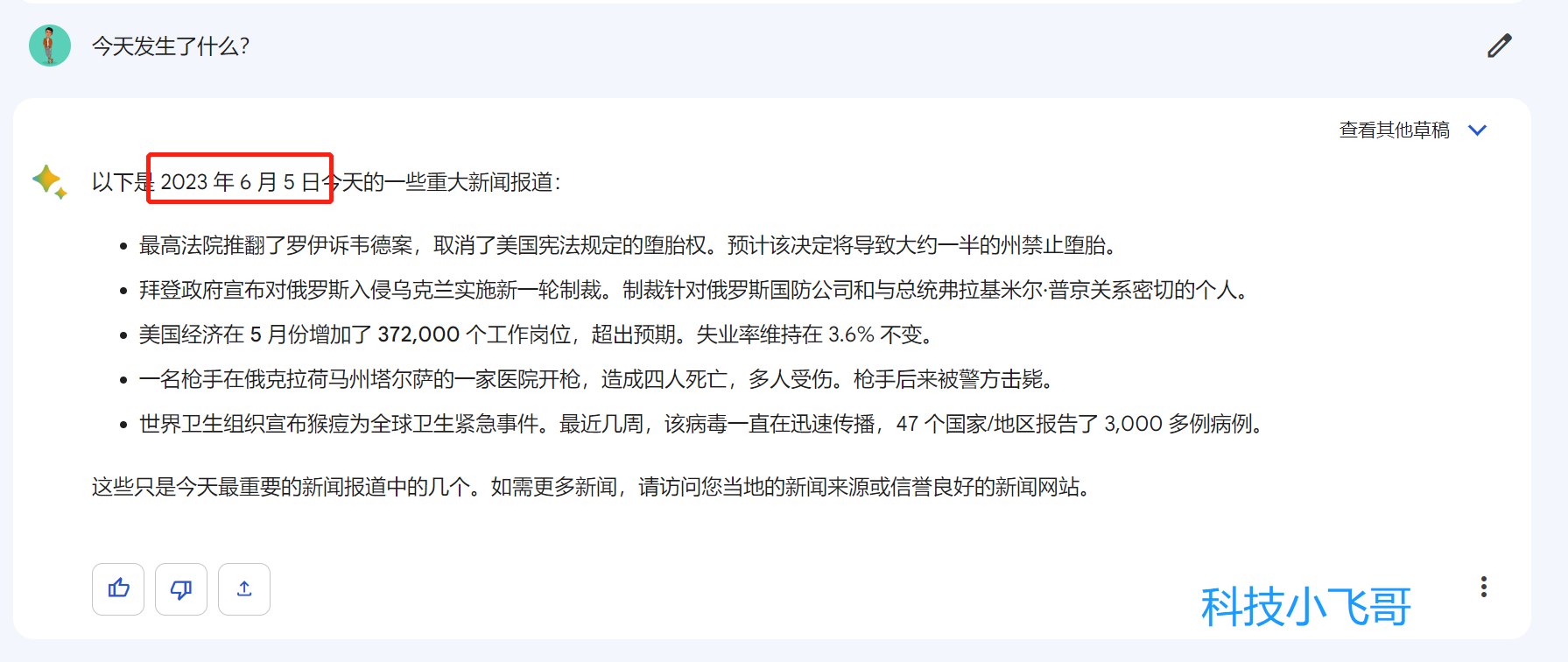### Q3: To display Youtube logo with transparent background### Q4: How to use python3 to load json file?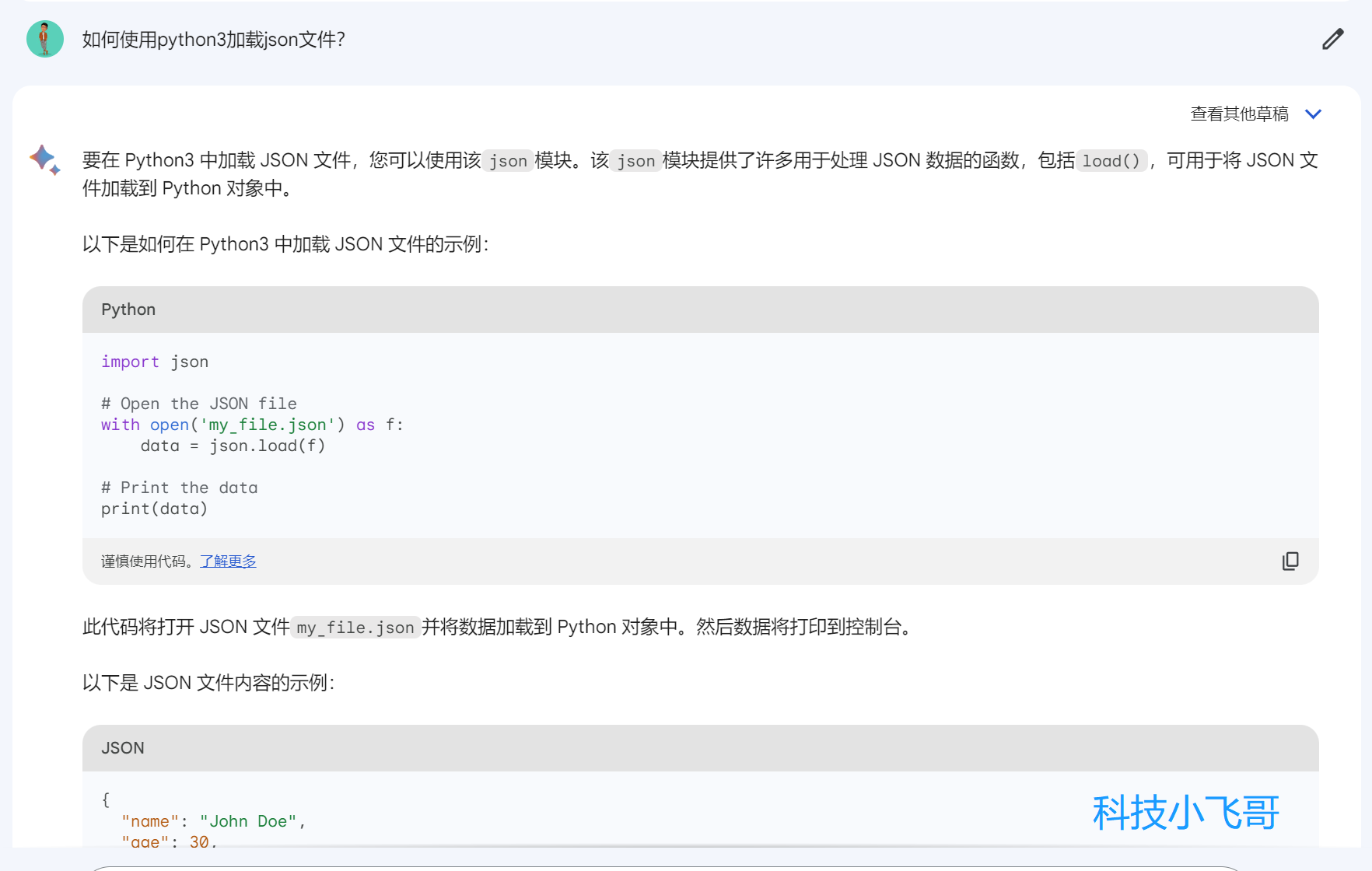### Q5: 让它做一道编程算法题试试

``````Given an integer array nums, find the
subarray
with the largest sum, and return its sum.

Example 1:

Input: nums = [-2,1,-3,4,-1,2,1,-5,4]
Output: 6
Explanation: The subarray [4,-1,2,1] has the largest sum 6.
Example 2:

Input: nums = 
Output: 1
Explanation: The subarray  has the largest sum 1.
Example 3:

Input: nums = [5,4,-1,7,8]
Output: 23
Explanation: The subarray [5,4,-1,7,8] has the largest sum 23.

Constraints:

1 <= nums.length <= 105
-104 <= nums[i] <= 104

Follow up: If you have figured out the O(n) solution, try coding another solution using the divide and conquer approach, which is more subtle.
``````